Скачать презентацию Inferring Disjunctive Postconditions Corneliu Popeea and Wei-Ngan Chin

8e8380ebf86c8cb3d3e9b533508cdfd1.ppt

• Количество слайдов: 35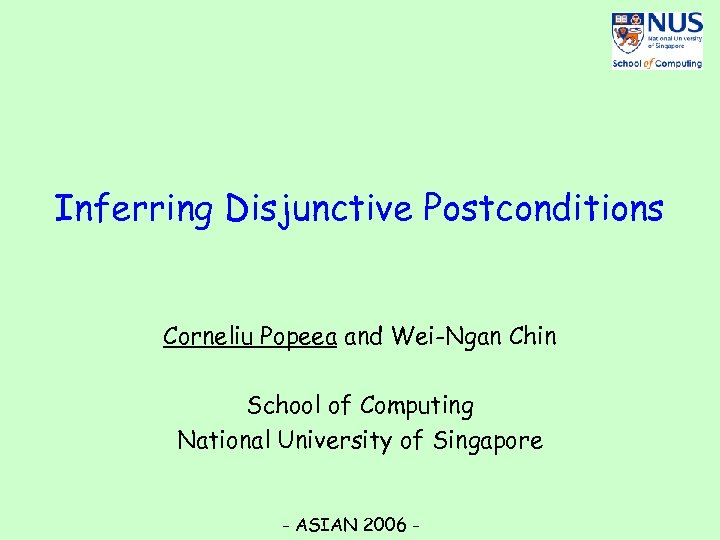Inferring Disjunctive Postconditions Corneliu Popeea and Wei-Ngan Chin School of Computing National University of Singapore - ASIAN 2006 -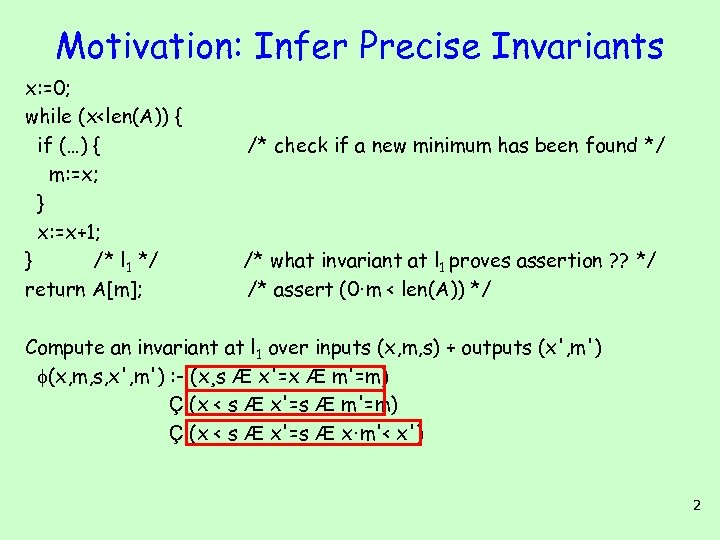Motivation: Infer Precise Invariants x: =0; while (x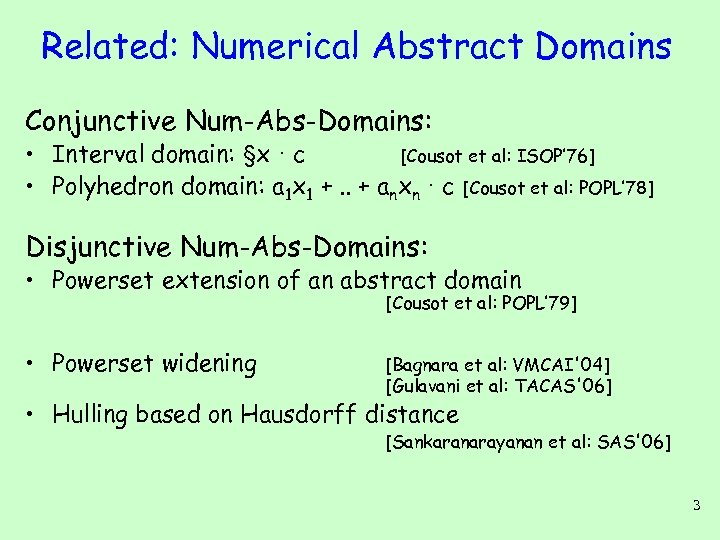Related: Numerical Abstract Domains Conjunctive Num-Abs-Domains: • Interval domain: §x · c [Cousot et al: ISOP’ 76] • Polyhedron domain: a 1 x 1 +. . + anxn · c [Cousot et al: POPL’ 78] Disjunctive Num-Abs-Domains: • Powerset extension of an abstract domain [Cousot et al: POPL’ 79] • Powerset widening [Bagnara et al: VMCAI'04] [Gulavani et al: TACAS'06] • Hulling based on Hausdorff distance [Sankaranarayanan et al: SAS'06] 3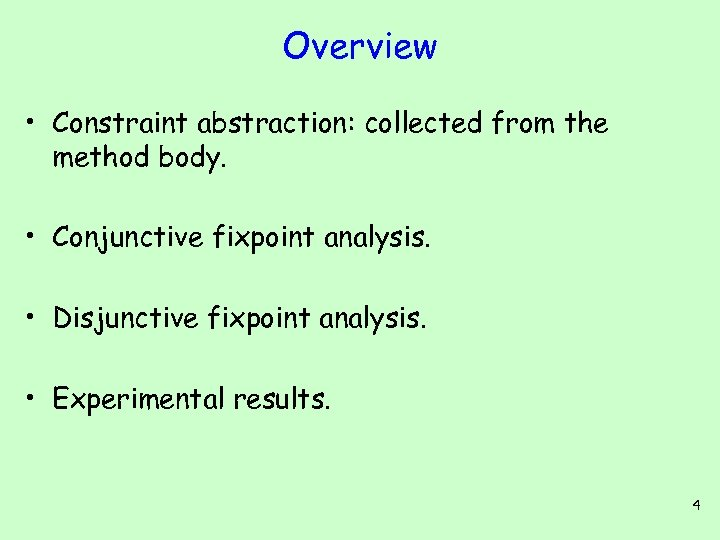Overview • Constraint abstraction: collected from the method body. • Conjunctive fixpoint analysis. • Disjunctive fixpoint analysis. • Experimental results. 4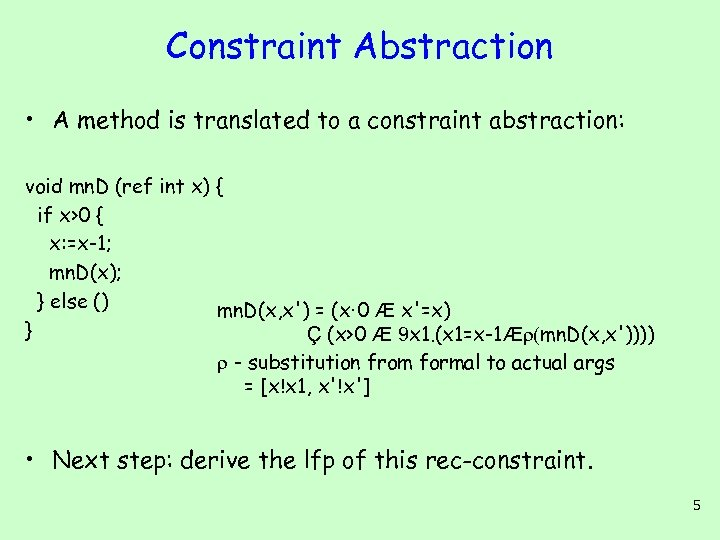Constraint Abstraction • A method is translated to a constraint abstraction: void mn. D (ref int x) { if x>0 { x: =x-1; mn. D(x); } else () mn. D(x, x') = (x· 0 Æ x'=x) } Ç (x>0 Æ 9 x 1. (x 1=x-1Æ (mn. D(x, x')))) - substitution from formal to actual args = [x!x 1, x'!x'] • Next step: derive the lfp of this rec-constraint. 5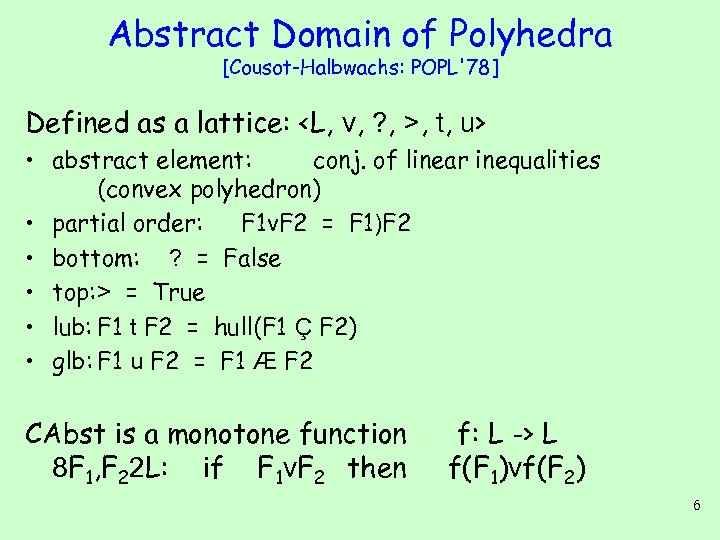Abstract Domain of Polyhedra [Cousot-Halbwachs: POPL'78] Defined as a lattice: , t, u> • abstract element: conj. of linear inequalities (convex polyhedron) • partial order: F 1 v. F 2 = F 1)F 2 • bottom: ? = False • top: > = True • lub: F 1 t F 2 = hull(F 1 Ç F 2) • glb: F 1 u F 2 = F 1 Æ F 2 CAbst is a monotone function 8 F 1, F 22 L: if F 1 v. F 2 then f: L -> L f(F 1)vf(F 2) 6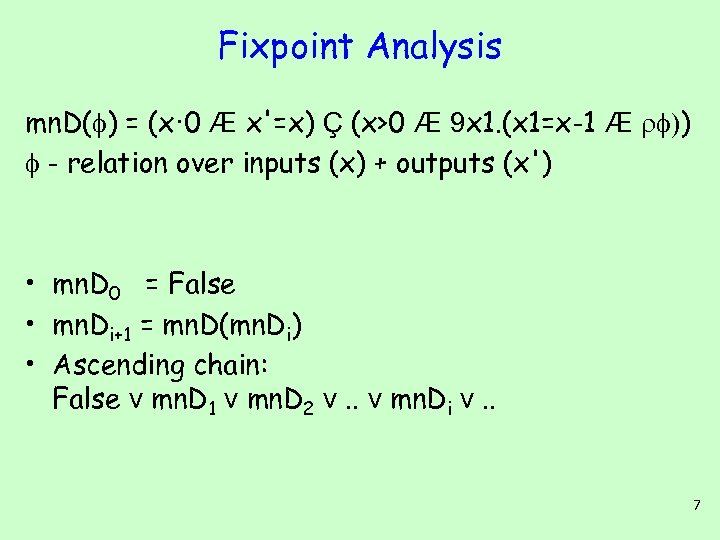Fixpoint Analysis mn. D( ) = (x· 0 Æ x'=x) Ç (x>0 Æ 9 x 1. (x 1=x-1 Æ )) - relation over inputs (x) + outputs (x') • mn. D 0 = False • mn. Di+1 = mn. D(mn. Di) • Ascending chain: False v mn. D 1 v mn. D 2 v. . v mn. Di v. . 7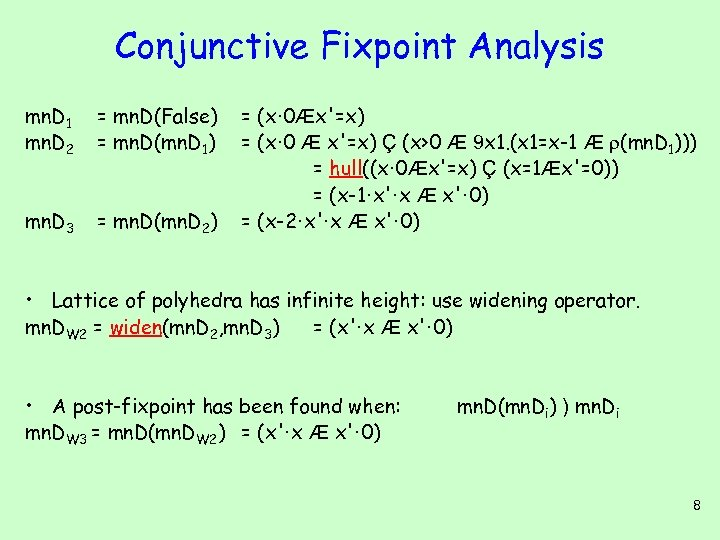Conjunctive Fixpoint Analysis mn. D 1 mn. D 2 = mn. D(False) = mn. D(mn. D 1) mn. D 3 = mn. D(mn. D 2) = (x· 0Æx'=x) = (x· 0 Æ x'=x) Ç (x>0 Æ 9 x 1. (x 1=x-1 Æ (mn. D 1))) = hull((x· 0Æx'=x) Ç (x=1Æx'=0)) = (x-1·x'·x Æ x'· 0) = (x-2·x'·x Æ x'· 0) • Lattice of polyhedra has infinite height: use widening operator. mn. DW 2 = widen(mn. D 2, mn. D 3) = (x'·x Æ x'· 0) • A post-fixpoint has been found when: mn. DW 3 = mn. D(mn. DW 2) = (x'·x Æ x'· 0) mn. D(mn. Di) ) mn. Di 8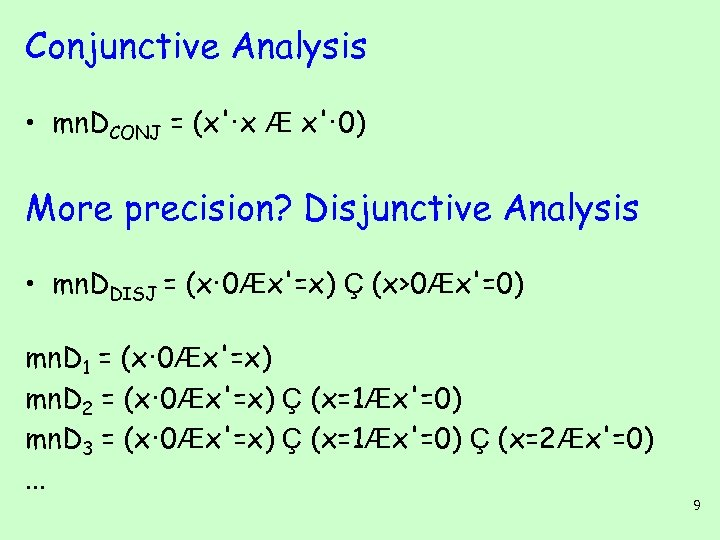Conjunctive Analysis • mn. DCONJ = (x'·x Æ x'· 0) More precision? Disjunctive Analysis • mn. DDISJ = (x· 0Æx'=x) Ç (x>0Æx'=0) mn. D 1 = (x· 0Æx'=x) mn. D 2 = (x· 0Æx'=x) Ç (x=1Æx'=0) mn. D 3 = (x· 0Æx'=x) Ç (x=1Æx'=0) Ç (x=2Æx'=0). . . 9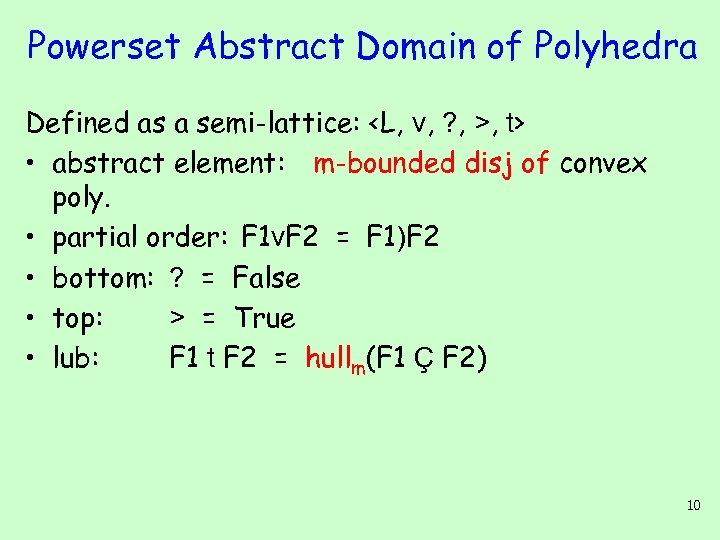Powerset Abstract Domain of Polyhedra Defined as a semi-lattice: , t> • abstract element: m-bounded disj of convex poly. • partial order: F 1 v. F 2 = F 1)F 2 • bottom: ? = False • top: > = True • lub: F 1 t F 2 = hullm(F 1 Ç F 2) 10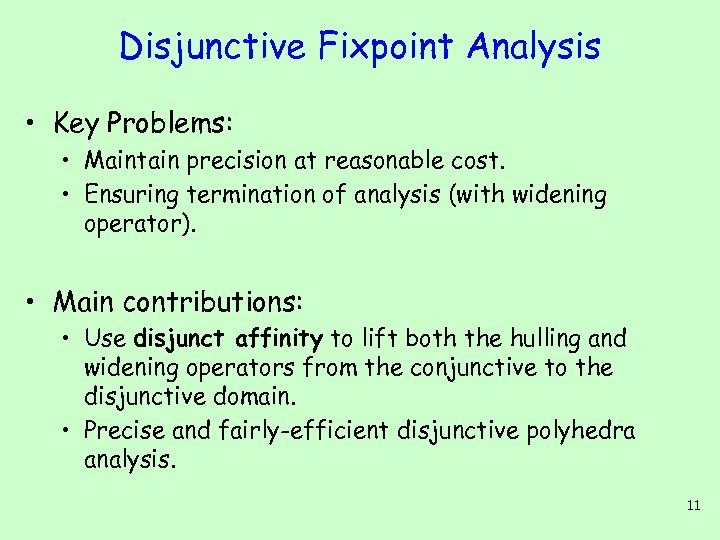Disjunctive Fixpoint Analysis • Key Problems: • Maintain precision at reasonable cost. • Ensuring termination of analysis (with widening operator). • Main contributions: • Use disjunct affinity to lift both the hulling and widening operators from the conjunctive to the disjunctive domain. • Precise and fairly-efficient disjunctive polyhedra analysis. 11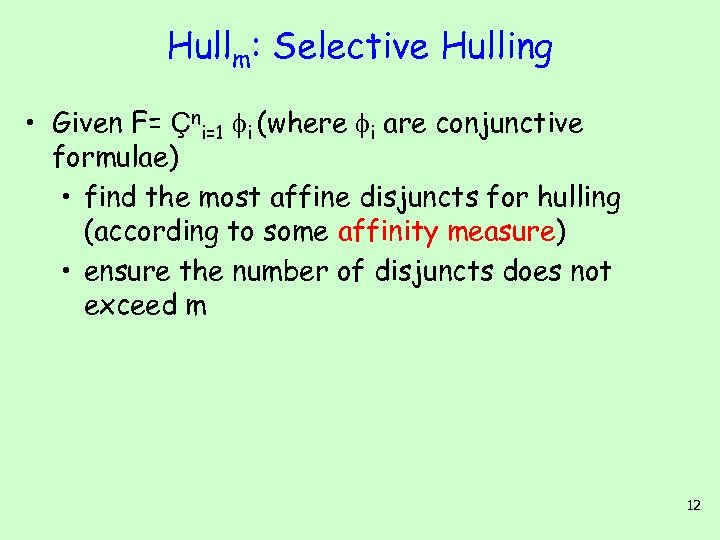Hullm: Selective Hulling • Given F= Çni=1 i (where i are conjunctive formulae) • find the most affine disjuncts for hulling (according to some affinity measure) • ensure the number of disjuncts does not exceed m 12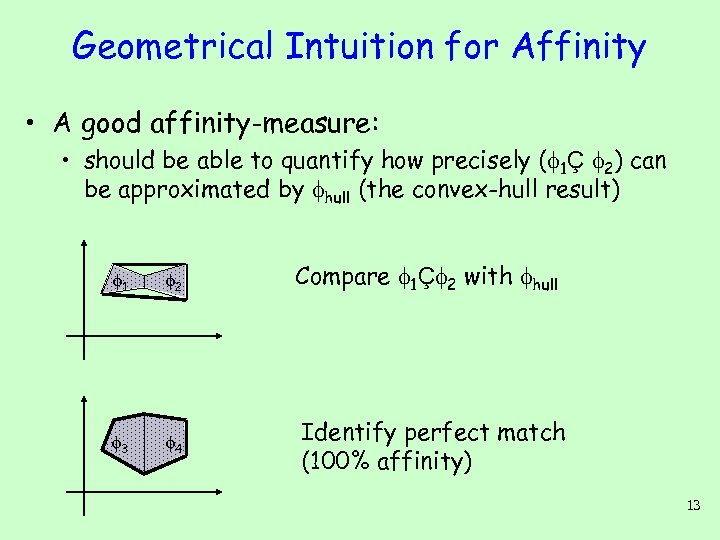Geometrical Intuition for Affinity • A good affinity-measure: • should be able to quantify how precisely ( 1Ç 2) can be approximated by hull (the convex-hull result) 1 2 3 4 Compare 1Ç 2 with hull Identify perfect match (100% affinity) 13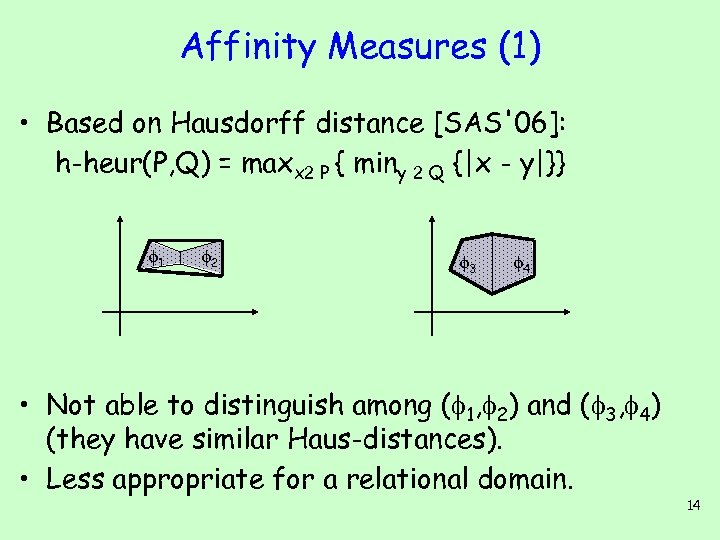Affinity Measures (1) • Based on Hausdorff distance [SAS'06]: h-heur(P, Q) = maxx 2 P { miny 2 Q {|x - y|}} 1 2 3 4 • Not able to distinguish among ( 1, 2) and ( 3, 4) (they have similar Haus-distances). • Less appropriate for a relational domain. 14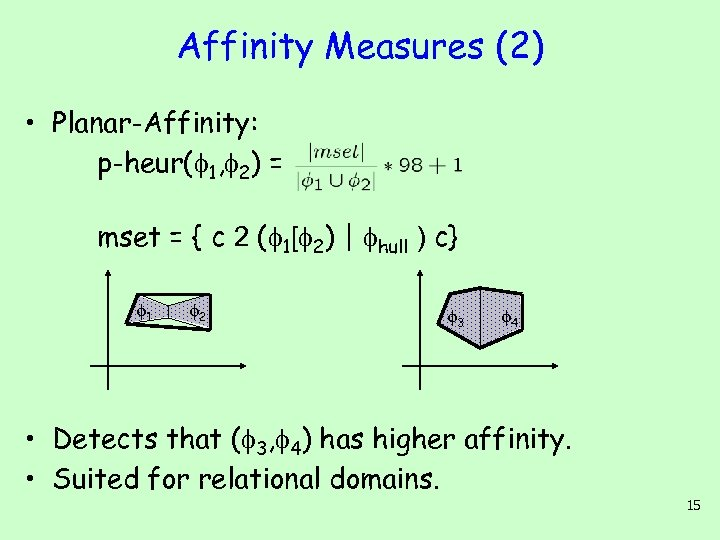Affinity Measures (2) • Planar-Affinity: p-heur( 1, 2) = mset = { c 2 ( 1[ 2) | hull ) c} 1 2 3 4 • Detects that ( 3, 4) has higher affinity. • Suited for relational domains. 15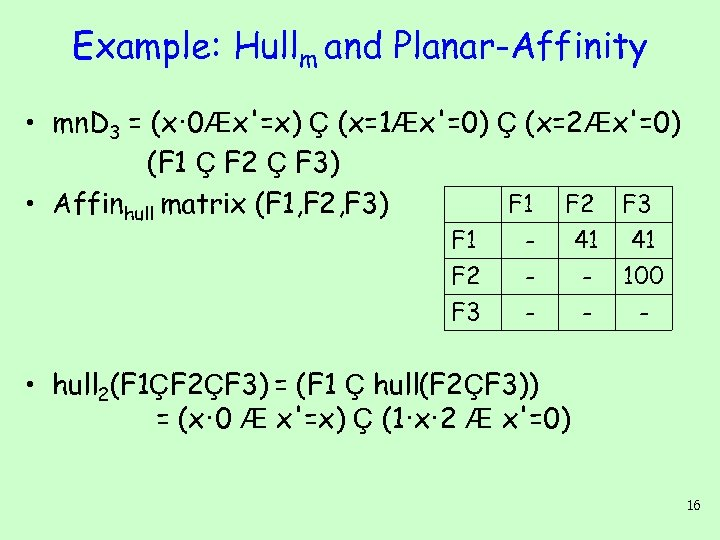Example: Hullm and Planar-Affinity • mn. D 3 = (x· 0Æx'=x) Ç (x=1Æx'=0) Ç (x=2Æx'=0) (F 1 Ç F 2 Ç F 3) F 1 F 2 F 3 • Affinhull matrix (F 1, F 2, F 3) F 1 F 2 F 3 - 41 100 - • hull 2(F 1ÇF 2ÇF 3) = (F 1 Ç hull(F 2ÇF 3)) = (x· 0 Æ x'=x) Ç (1·x· 2 Æ x'=0) 16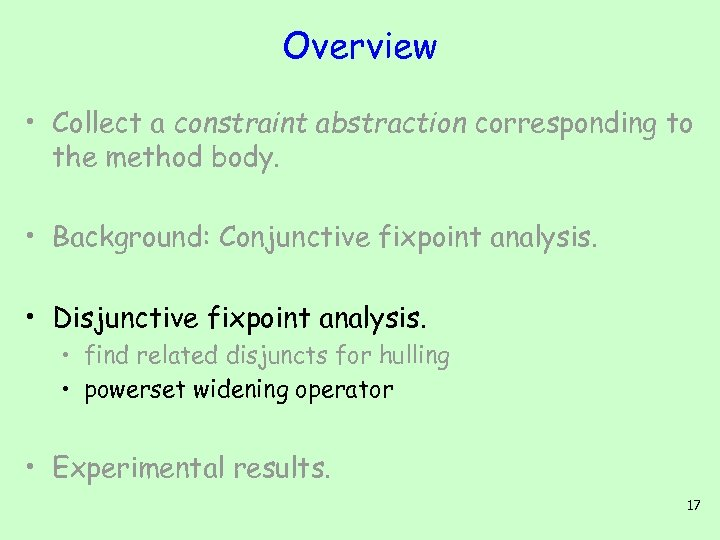Overview • Collect a constraint abstraction corresponding to the method body. • Background: Conjunctive fixpoint analysis. • Disjunctive fixpoint analysis. • find related disjuncts for hulling • powerset widening operator • Experimental results. 17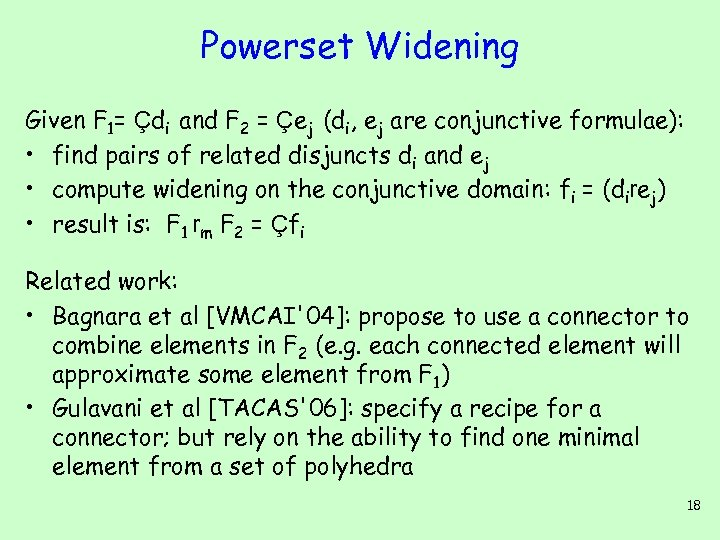Powerset Widening Given F 1= Çdi and F 2 = Çej (di, ej are conjunctive formulae): • find pairs of related disjuncts di and ej • compute widening on the conjunctive domain: fi = (direj) • result is: F 1 rm F 2 = Çfi Related work: • Bagnara et al [VMCAI'04]: propose to use a connector to combine elements in F 2 (e. g. each connected element will approximate some element from F 1) • Gulavani et al [TACAS'06]: specify a recipe for a connector; but rely on the ability to find one minimal element from a set of polyhedra 18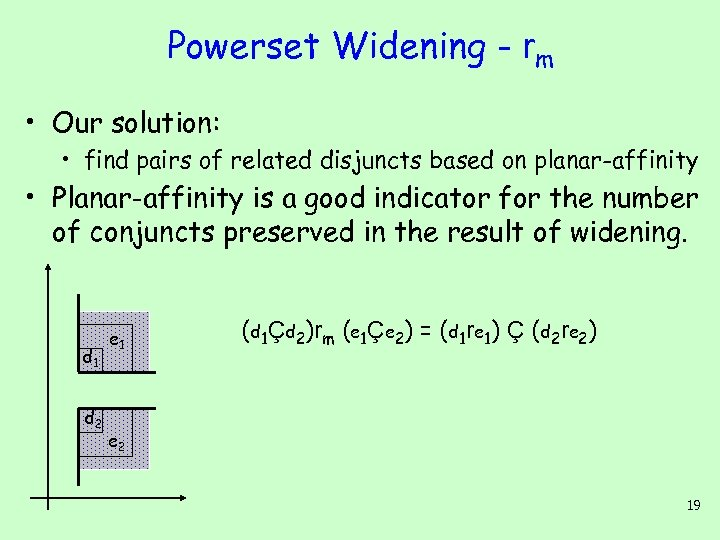Powerset Widening - rm • Our solution: • find pairs of related disjuncts based on planar-affinity • Planar-affinity is a good indicator for the number of conjuncts preserved in the result of widening. d 1 d 2 e 1 (d 1Çd 2)rm (e 1Çe 2) = (d 1 re 1) Ç (d 2 re 2) e 2 19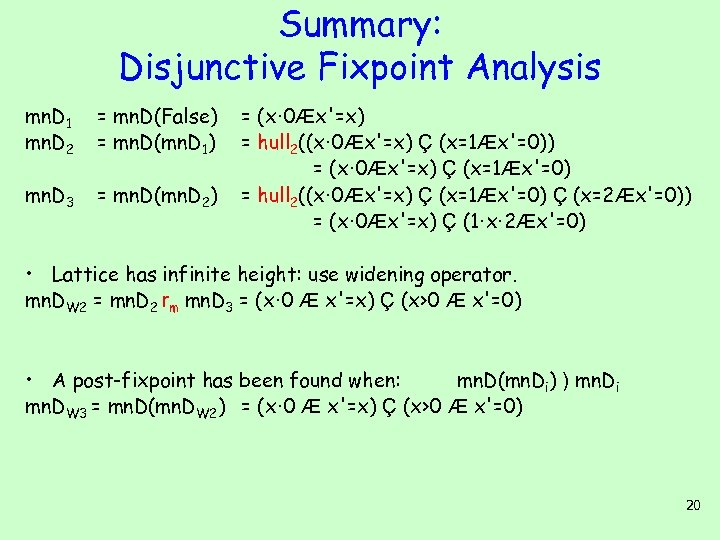Summary: Disjunctive Fixpoint Analysis mn. D 1 mn. D 2 = mn. D(False) = mn. D(mn. D 1) mn. D 3 = mn. D(mn. D 2) = (x· 0Æx'=x) = hull 2((x· 0Æx'=x) Ç (x=1Æx'=0)) = (x· 0Æx'=x) Ç (x=1Æx'=0) = hull 2((x· 0Æx'=x) Ç (x=1Æx'=0) Ç (x=2Æx'=0)) = (x· 0Æx'=x) Ç (1·x· 2Æx'=0) • Lattice has infinite height: use widening operator. mn. DW 2 = mn. D 2 rm mn. D 3 = (x· 0 Æ x'=x) Ç (x>0 Æ x'=0) • A post-fixpoint has been found when: mn. D(mn. Di) ) mn. Di mn. DW 3 = mn. D(mn. DW 2) = (x· 0 Æ x'=x) Ç (x>0 Æ x'=0) 20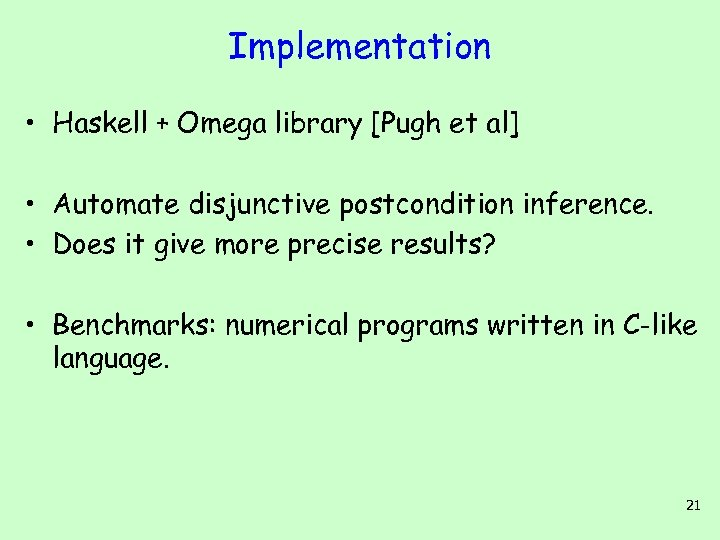Implementation • Haskell + Omega library [Pugh et al] • Automate disjunctive postcondition inference. • Does it give more precise results? • Benchmarks: numerical programs written in C-like language. 21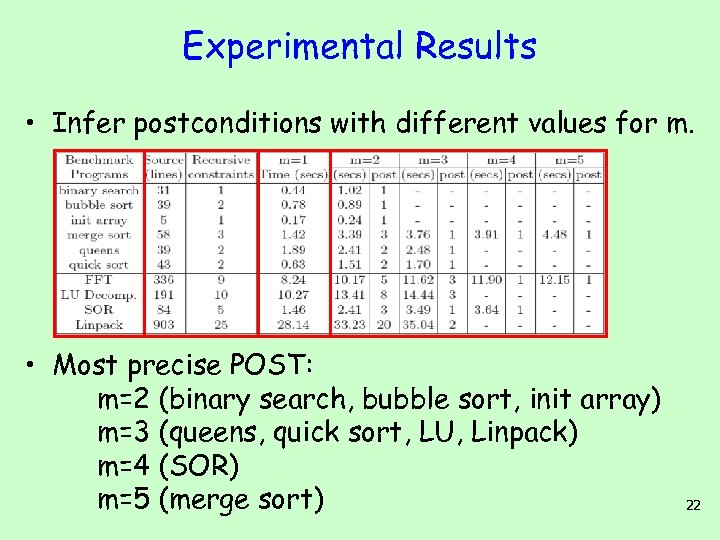Experimental Results • Infer postconditions with different values for m. • Most precise POST: m=2 (binary search, bubble sort, init array) m=3 (queens, quick sort, LU, Linpack) m=4 (SOR) m=5 (merge sort) 22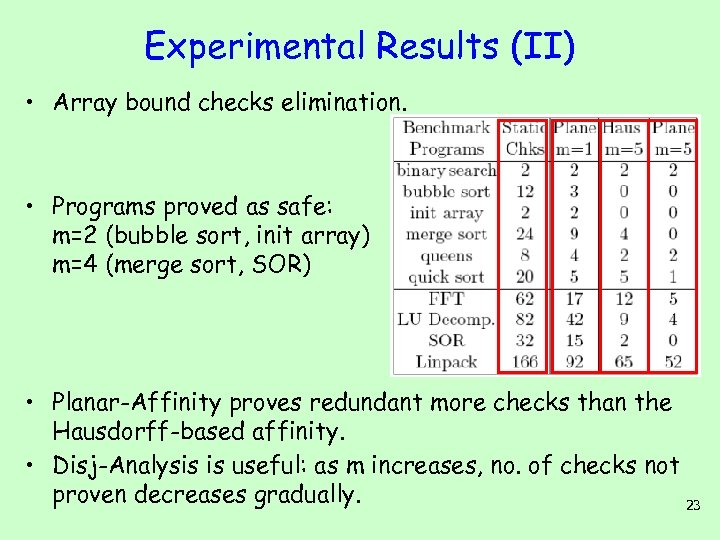Experimental Results (II) • Array bound checks elimination. • Programs proved as safe: m=2 (bubble sort, init array) m=4 (merge sort, SOR) • Planar-Affinity proves redundant more checks than the Hausdorff-based affinity. • Disj-Analysis is useful: as m increases, no. of checks not proven decreases gradually. 23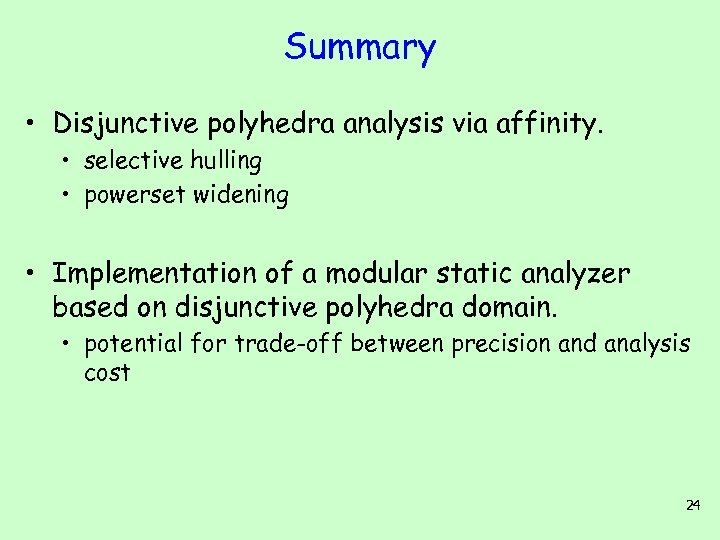Summary • Disjunctive polyhedra analysis via affinity. • selective hulling • powerset widening • Implementation of a modular static analyzer based on disjunctive polyhedra domain. • potential for trade-off between precision and analysis cost 24The End 25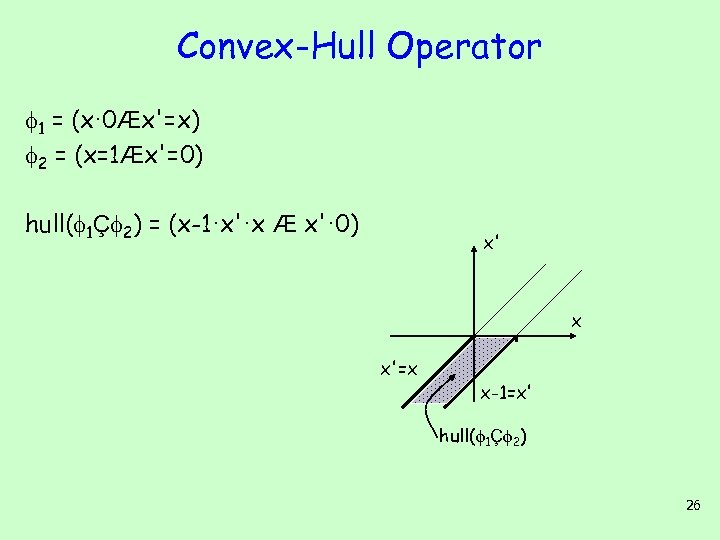Convex-Hull Operator 1 = (x· 0Æx'=x) 2 = (x=1Æx'=0) hull( 1Ç 2) = (x-1·x'·x Æ x'· 0) x' x x'=x x-1=x' hull( 1Ç 2) 26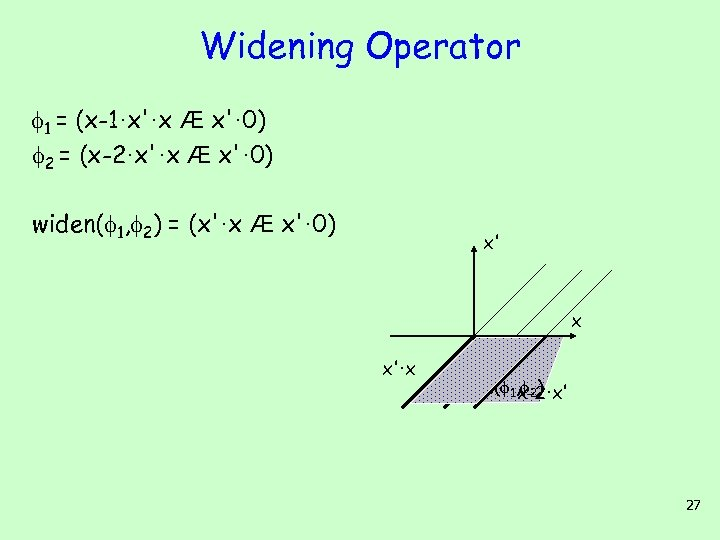Widening Operator 1 = (x-1·x'·x Æ x'· 0) 2 = (x-2·x'·x Æ x'· 0) widen( 1, 2) = (x'·x Æ x'· 0) x' x x'·x widen( 1, 2) x-1·x' x-2·x' 27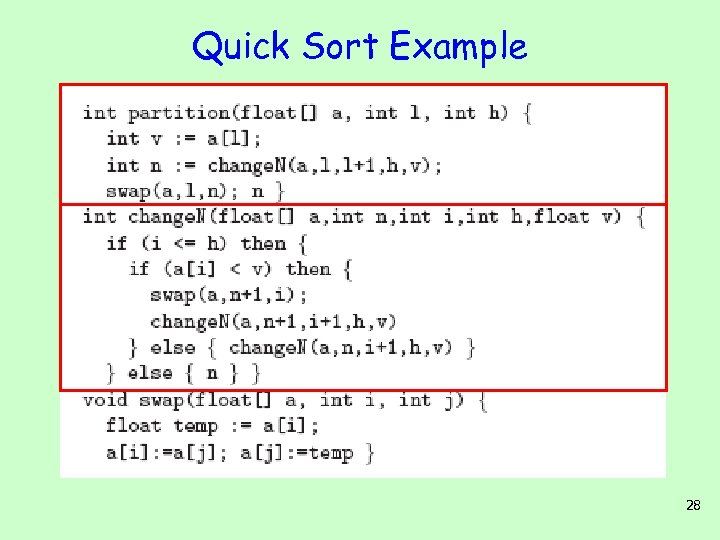Quick Sort Example 28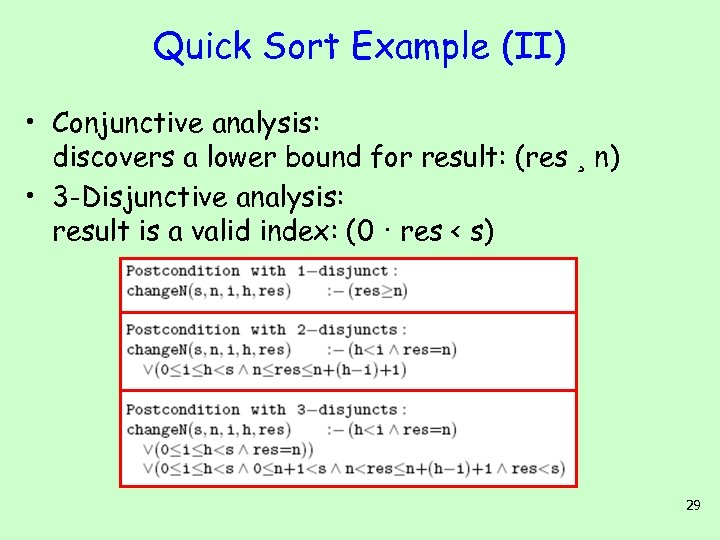Quick Sort Example (II) • Conjunctive analysis: discovers a lower bound for result: (res ¸ n) • 3 -Disjunctive analysis: result is a valid index: (0 · res < s) 29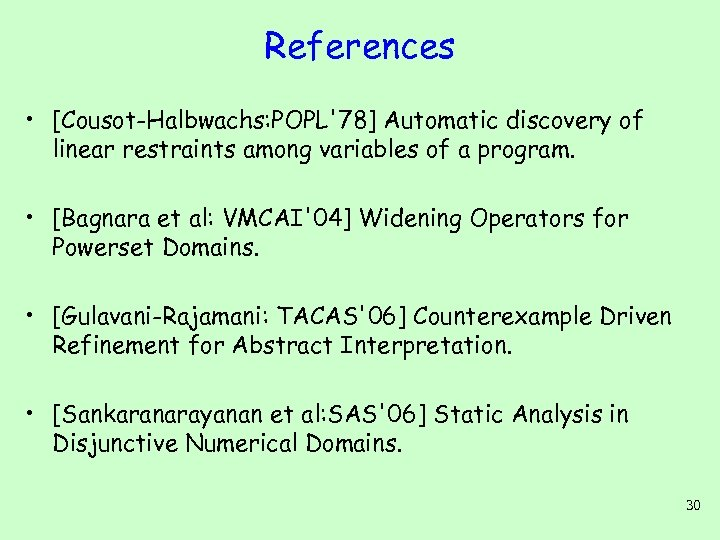References • [Cousot-Halbwachs: POPL'78] Automatic discovery of linear restraints among variables of a program. • [Bagnara et al: VMCAI'04] Widening Operators for Powerset Domains. • [Gulavani-Rajamani: TACAS'06] Counterexample Driven Refinement for Abstract Interpretation. • [Sankaranarayanan et al: SAS'06] Static Analysis in Disjunctive Numerical Domains. 30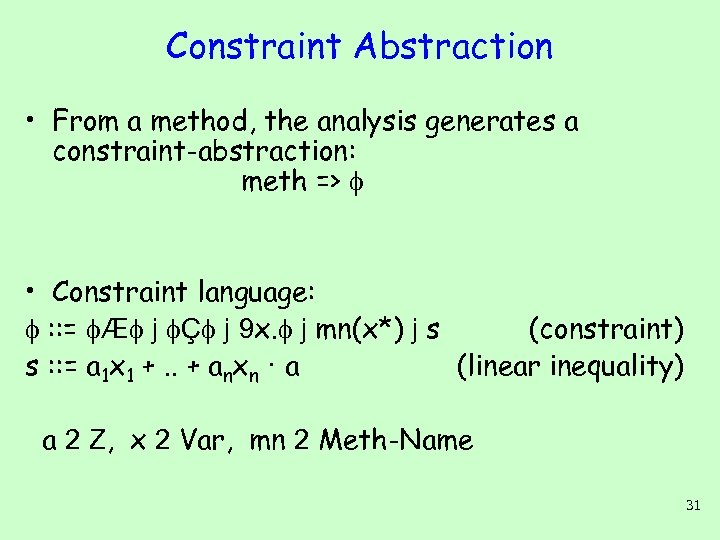Constraint Abstraction • From a method, the analysis generates a constraint-abstraction: meth => • Constraint language: : : = Æ j Ç j 9 x. j mn(x*) j s (constraint) s : : = a 1 x 1 +. . + anxn · a (linear inequality) a 2 Z, x 2 Var, mn 2 Meth-Name 31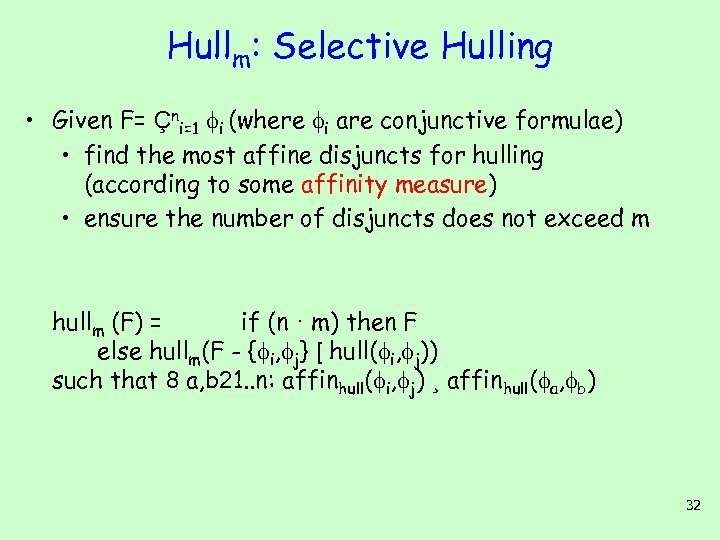Hullm: Selective Hulling • Given F= Çni=1 i (where i are conjunctive formulae) • find the most affine disjuncts for hulling (according to some affinity measure) • ensure the number of disjuncts does not exceed m hullm (F) = if (n · m) then F else hullm(F - { i, j} [ hull( i, j)) such that 8 a, b 21. . n: affinhull( i, j) ¸ affinhull( a, b) 32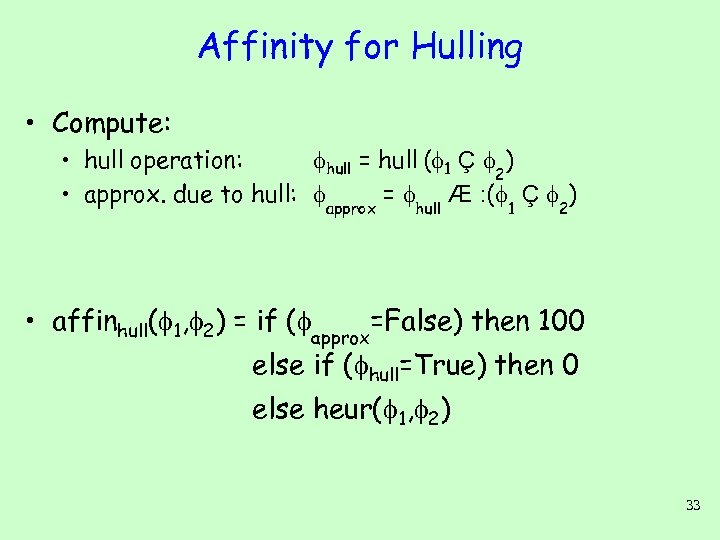Affinity for Hulling • Compute: • hull operation: hull = hull ( 1 Ç 2) • approx. due to hull: approx = hull Æ : ( 1 Ç 2) • affinhull( 1, 2) = if ( approx=False) then 100 else if ( hull=True) then 0 else heur( 1, 2) 33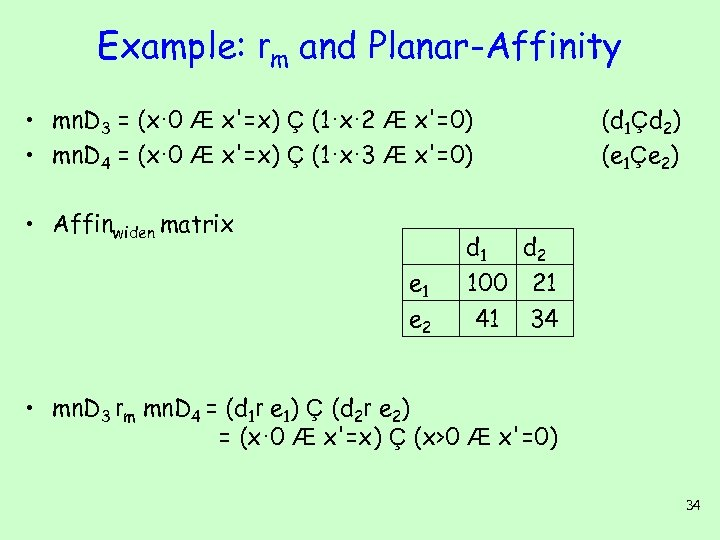Example: rm and Planar-Affinity • mn. D 3 = (x· 0 Æ x'=x) Ç (1·x· 2 Æ x'=0) • mn. D 4 = (x· 0 Æ x'=x) Ç (1·x· 3 Æ x'=0) • Affinwiden matrix e 1 e 2 (d 1Çd 2) (e 1Çe 2) d 1 d 2 100 21 41 34 • mn. D 3 rm mn. D 4 = (d 1 r e 1) Ç (d 2 r e 2) = (x· 0 Æ x'=x) Ç (x>0 Æ x'=0) 34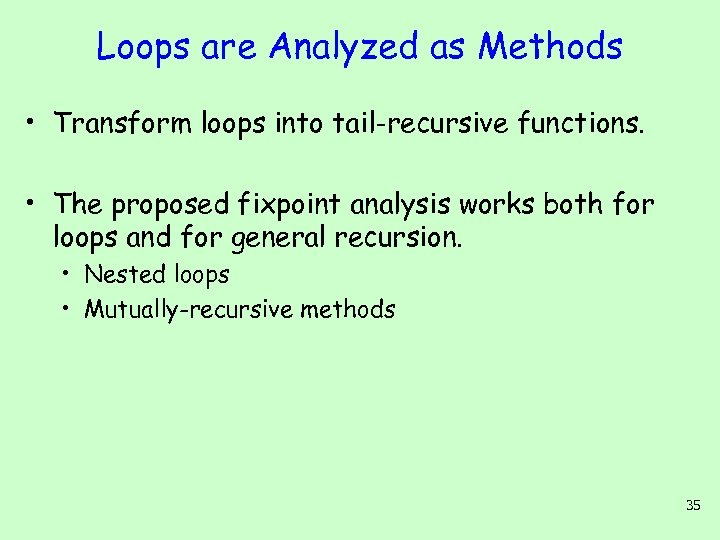Loops are Analyzed as Methods • Transform loops into tail-recursive functions. • The proposed fixpoint analysis works both for loops and for general recursion. • Nested loops • Mutually-recursive methods 35